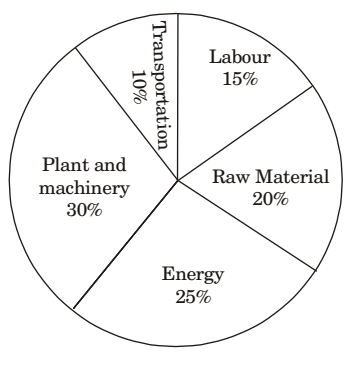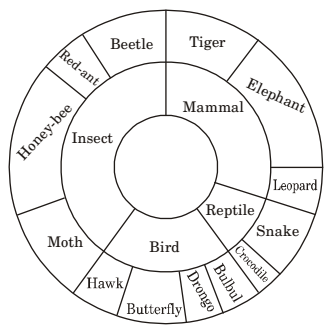## Numerical Ability

#### Numerical Ability

1. Let f[x, y) = xn ym = P. If x is doubled and y is halved, the new value of f is

1.  P' = 2nXn1m ym 7

= 2n - mXnYm = 2n - m P

##### Correct Option: A

 P' = 2nXn1m ym 7

= 2n - mXnYm = 2n - m P

1. A firm producing air purifiers sold 200 units in 2012. The following pie chart presents the share of raw material, labour, energy, plant & machinery, and transportation costs in the total manufacturing cost of the firm in 2012. The expenditure on labour in 2012 is Rs. 4,50,000. In 2013, the raw material expenses increased by 30% and all other expenses increased by 20%. If the company registered a profit of Rs. 10 lakhs in 2012, at what price (in Rs.) was each air purifier sold?1. Total expenditure

 = 15 x = 4,50,000 100

x = 3 × 106
Profit = 10 lakhs
So, total selling price = 40,00,000 ...(1)
Total purifies = 200 ...(2)
S.P. of each purifier = (1)/(2) = 20,000

##### Correct Option: C

Total expenditure

 = 15 x = 4,50,000 100

x = 3 × 106
Profit = 10 lakhs
So, total selling price = 40,00,000 ...(1)
Total purifies = 200 ...(2)
S.P. of each purifier = (1)/(2) = 20,000

1. A man can row at 8 km per hour in still water. If it takes him thrice as long to row upstream, as to row downstream, then find the stream velocity in km per hour.

1. Speed of man = 8;
Left distance = d
Time taken = 8/d
Upstream: Speed of stream = s
⇒ speed upstream = S’ = (8 – s)

 t' =d8 - s

Downstream:
 Given speed downstream = t" = d 8 + s

⇒ 3t' = t"
 ⇒ 3d = d 8 - s 8 + s

⇒ s = 4 Km/hr

##### Correct Option: A

Speed of man = 8;
Left distance = d
Time taken = 8/d
Upstream: Speed of stream = s
⇒ speed upstream = S’ = (8 – s)

 t' =d8 - s

Downstream:
 Given speed downstream = t" = d 8 + s

⇒ 3t' = t"
 ⇒ 3d = d 8 - s 8 + s

⇒ s = 4 Km/hr

1. The multi-level hierarchical pie chart shows the population of animals in a reserve forest. The correct conclusions from this information are:(i) Butterflies are birds
(ii) There are more tigers in this forest than red ants
(iii) All reptiles in this forest are either snakes or crocodiles
(iv) Elephants are the largest mammals in this forest

1. It is not mentioned that elephant is the largest animal.

##### Correct Option: D

It is not mentioned that elephant is the largest animal.

1. Find the next term in the sequence: 7 G, 11 K, 13M, _______.

1. In 7G - G is the 7th alphabet
11K - K is the 11th alphabet
13M - M is the 13th alphabet
Also, 7, 11, 13 are prime numbers, so next prime number will be 17 with correct answer as 17Q.

##### Correct Option: B

In 7G - G is the 7th alphabet
11K - K is the 11th alphabet
13M - M is the 13th alphabet
Also, 7, 11, 13 are prime numbers, so next prime number will be 17 with correct answer as 17Q.